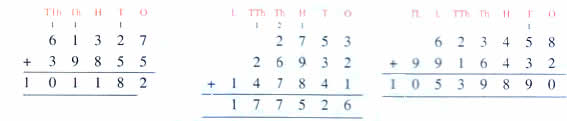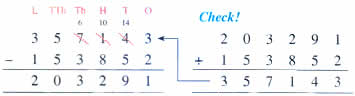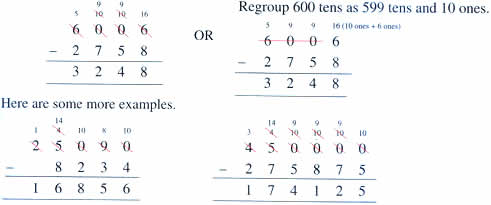Wednesday , October 5 2022# NCERT 5th Class (CBSE) Mathematics: The Four Operations And Their Application

## ADDITION AND SUBTRACTION

### Properties of Addition:

1. When you change the order of the addends, the sum does not change.
2. The sum of any number and zero is that same number.

Adding large numbers is the same as adding smaller numbers. You start from the ones place and regroup (carry over) if necessary.### Properties of Subtraction:

1. Any number subtracted from itself is zero.
2. When you subtract zero from a number you get the number itself.

Subtracting large numbers is the same as subtracting smaller numbers. Write them in the correct place and start subtracting from the ones place. Regroup if necessary.### Watch out for the zeros!## चुनौती हिमालय की 5th NCERT CBSE Hindi Rimjhim Ch 18

चुनौती हिमालय की 5th Class NCERT CBSE Hindi Book Rimjhim Chapter 18 प्रश्न: लद्दाख जम्मू-कश्मीर राज्य में …